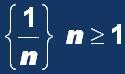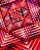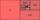# Five members

Write first 5 members geometric sequence and determine whether it is increasing or decreasing:
a1 = 3
q = -2

Result

a1 =  3
a2 =  -6
a3 =  12
a4 =  -24
a5 =  48

a5 =

### Step-by-step explanation:

${a}_{1}=3$
${a}_{2}=3\cdot -2=-6$
${a}_{3}=3\cdot -{2}^{2}=12$
${a}_{4}=3\cdot -{2}^{3}=-24$
${a}_{5}=3\cdot -{2}^{4}=48$Did you find an error or inaccuracy? Feel free to write us. Thank you!## Related math problems and questions:

• Geometric sequence 3In geometric sequence is a8 = 312500; a11= 39062500; sn=1953124. Calculate the first item a1, quotient q, and n - number of members by their sum s_n.
• Geometric sequence 5About members of geometric sequence we know: ? ? Calculate a1 (first member) and q (common ratio or q-coefficient)
• SequenceWrite the first 6 members of this sequence: a1 = 5 a2 = 7 an+2 = an+1 +2 an
• Geometric sequence 4It is given geometric sequence a3 = 7 and a12 = 3. Calculate s23 (= sum of the first 23 members of the sequence).
• SequenceWrite the first 7 members of an arithmetic sequence: a1=-3, d=6.
• Geometric sequenceIn the geometric sequence is a4 = 20 a9 = -160. Calculate the first member a1 and quotient q.
• Sequence - 5 membersWrite first five members of the sequence ?
• GP - 8 itemsDetermine the first eight members of a geometric progression if a9=512, q=2
• Tenth memberCalculate the tenth member of geometric sequence when given: a1=1/2 and q=2
• Five elementThe geometric sequence is given by quotient q = 1/2 and the sum of the first six members S6 = 63. Find the fifth element a5.
• GP membersThe geometric sequence has 10 members. The last two members are 2 and -1. Which member is -1/16?
• Geometric progressionIn geometric progression, a1 = 7, q = 5. Find the condition for n to sum first n members is sn≤217.
• Sequence 3Write the first 5 members of an arithmetic sequence: a4=-35, a11=-105.
• AS sequenceIn an arithmetic sequence is given the difference d = -3 and a71 = 455. a) Determine the value of a62 b) Determine the sum of 71 members.
• Sequence 2Write the first 5 members of an arithmetic sequence a11=-14, d=-1
• Gp - 80One of the first four members of a geometric progression is 80. Find its if we know that the fourth member is nine times greater than the second.
• GP - three membersThe second and third of a geometric progression are 24 and 12(c+1), respectively, given that the sum of the first three terms of progression is 76. determine the value of c.